# Learn How to Use One-Dimensional Array in C++

###### Arrays in C++

This lesson will help you understand One-Dimensional Array in C++ Programming with easy-to-follow examples and explanations. You'll be a pro in no time!

## What is One Dimensional Array (1D Array) in C++

A One-Dimensional Array in C++ programming is a special type of variable that can store multiple values of only a single data type such as int, float, double, char, structure, pointer, etc. at a contagious location in computer memory. Here contagious location means at a fixed gap in computer memory.

A One-Dimensional Array is also known as 1D Array.

Suppose we want to store the age of 10 students. In that case, we have to declare 10 variables in our C++ program to store the age of 10 students.

Now here comes the use of a one-dimensional array. With the help of 1D Array, we will declare a single variable in our C++ program that can store the age of 10 students at a time.### Declaration Syntax of a One Dimensional Array in C++

``datatype variable_name[size];``

Here, size is the number of elements we want to store in the array.

#### Example

``int a;``

Once we declare the 1D Array, it will look like as shown in the picture below: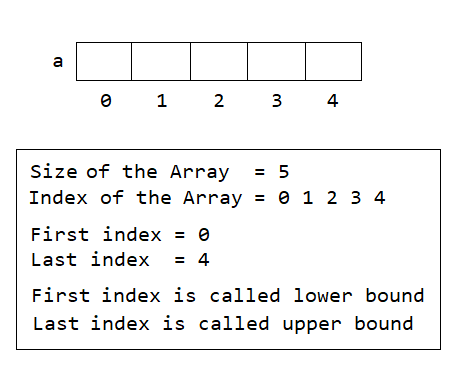In above image we can see that the name of the one dimensional array is a and it can store 5 integer numbers. Size of the array is 5. Index of the array is 0, 1, 2, 3 and 4.

The first index is called Lower Bound, and the last index is called an Upper Bound. Upper Bound of a one dimensional is always Size – 1.

## Declaration and Initialization of a One Dimensional Array in C++

In C++ programming a one dimensional array can be declared and initialized in several ways. Let’s see the different ways of initializing a 1D array.

#### Example 1: (with size mentioned)

``int a={12,18,6};``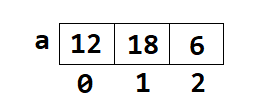#### Example 2: (without size mentioned)

``int a[]={7,12,9};``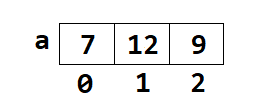#### Example 3: (1st cell contains the value 5 and, rest of the cells 0)

``int a={5};``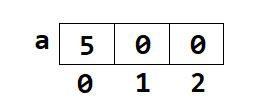#### Example 4: (All cell contains the value 0)

``int a={};``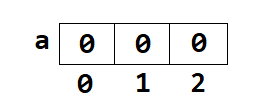## Store Numbers in a One Dimensional Array

To store the number in each cell of the array we can use the following syntax.

``array_name[index]=value;``

#### Example

``````a=26;
a=15;
a=34;
``````

## Access Numbers in a One Dimensional Array

We can access any number stored in a 1D array using the following syntax.

``array_name[index];``

#### Example

``cout<<a<<" "<<a<<" "<<a;``

#### Output

`26 15 34`

## Store and Access the Numbers in a 1D Array using Loops

We can also store as well as access the numbers in a 1D array using either for, while or do while loop. Let's see a few examples.

#### Example 1

Program to input 10 numbers in an array and display only the even numbers if present in the array.

``````#include <iostream>
#include <conio.h>

using namespace std;

int main()
{
int a, i;

cout<<"Enter 10 numbers\n";
for(i=0; i<10; i++)
{
cin>>a[i];
}

cout<<"List of even numbers\n";
for(i=0; i<10; i++)
{
if(a[i]%2==0)
{
cout<<a[i]<<" ";
}
}
return 0;
}``````

#### Output

```Enter 10 numbers
11
15
28
31
49
54
72
81
93
14
List of even numbers
28 54 72 14```

Here, you can see that we have run a for loop 10 times to store the user's input in the array. After that we have run another for loop 10 times to access each number from the array and print only the even numbers from it.

#### Example 2

Program to input 5 numbers in an array and print all the numbers from the backside of the array. Example: 12 18 16 Output: 16 18 12

``````#include <iostream>
#include <conio.h>

using namespace std;

int main()
{
int a, i;

cout<<"Enter 5 numbers\n";
for(i=0; i<5; i++)
{
cin>>a[i];
}

for(i=4; i>=0; i--)
{
cout<<a[i]<<" ";
}
return 0;
}``````

#### Output

```Enter 5 numbers
48
21
97
64
53
53 64 97 21 48```

Here, you can see that we have run a for loop 5 times to store the user's input in the array. After that we have run another for loop in reverse order to print all the numbers from the back side of the array.